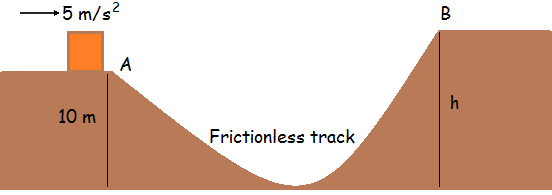Mechanics - 6A block starts with a velocity of $\text{5 m/s}$ at $A$, which is at a height $\text{10 m}$, ends just reaching $B$, which is at a height $h \text{ m}$. If all the surfaces are frictionless, find $h$. Take $g = \text{10 m/s}^2$.

Try my World of Physics to solve many problems like this one.

×